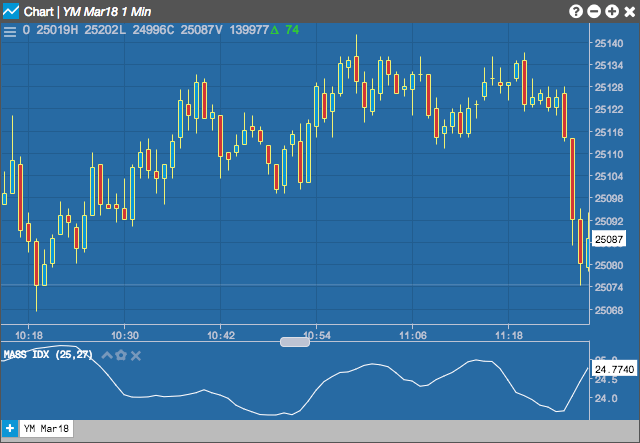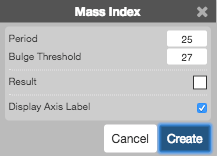Charts

# Mass Index (MI)

The Mass Index indicator, developed by Donald Dorsey, uses the bar range to identify trend reversals based on range expansions. It is more of a volatility indicator as it does not have a directional bias. It identifies trending and congession areas, which can occur before change in trend.## Configuration Options• Period: Number of bars to use in the calculations.
• Bulge Threshold: TBD
• Color Selectors: Colors to use for graph elements.
• Display Axis Label: Whether to display the most recent value on the Y axis.

## Formula

$EMA_{1} = 9-period\;EMA\;of\;the\;range\;(H-L)$

$DEMA_{1} = 9-period\;EMA_{1}$

$EMA Ratio = \frac{EMA_{1}}{DEMA_{1}}$

$MI = 25-period\;sum\;of\;the\;EMA\;Ratio$